# DAV Class 8 Maths Chapter 1 Worksheet 5 Solutions

The DAV Class 8 Maths Book Solutions and DAV Class 8 Maths Chapter 1 Worksheet 5 Solutions of Squares and Square Roots offer comprehensive answers to textbook questions.

## DAV Class 8 Maths Ch 1 WS 5 Solutions

Question 1.
Find the square root of the following fractions:

(i) $$\frac{324}{361}$$

(ii) $$\frac{441}{961}$$

(iii) $$5 \frac{19}{25}$$

(iv) $$21 \frac{51}{169}$$

(v) $$\frac{5625}{441}$$

(vi) $$7 \frac{18}{49}$$

(vii) $$23 \frac{394}{729}$$

(viii)$$35 \frac{85}{1444}$$

Solution:
(i) $$\sqrt{\frac{324}{361}}=\frac{\sqrt{324}}{\sqrt{361}}=\frac{\sqrt{18^2}}{\sqrt{19^2}}=\frac{18}{19}$$

(ii) $$\sqrt{\frac{441}{961}}=\frac{\sqrt{441}}{\sqrt{961}}=\frac{\sqrt{21^2}}{\sqrt{31^2}}=\frac{21}{31}$$

(iii) $$\sqrt{5 \frac{19}{25}}=\sqrt{\frac{144}{25}}=\frac{\sqrt{144}}{\sqrt{25}}$$
= $$\frac{\sqrt{12^2}}{\sqrt{5^2}}=\frac{12}{5}=2 \frac{2}{5}$$

(iv) $$\sqrt{21 \frac{51}{169}}=\sqrt{\frac{3600}{169}}=\frac{\sqrt{3600}}{\sqrt{169}}$$
= $$\frac{\sqrt{60^2}}{\sqrt{13^2}}=\frac{60}{13}=4 \frac{8}{13}$$

(v) $$\sqrt{\frac{5625}{441}}=\frac{\sqrt{5625}}{\sqrt{441}}=\frac{\sqrt{75^2}}{\sqrt{21^2}}$$
= $$\frac{75}{21}=3 \frac{12}{21}$$

(vi) $$\sqrt{7 \frac{18}{49}}=\sqrt{\frac{361}{49}}=\sqrt{\frac{19^2}{7^2}}$$
= $$\frac{19}{7}=2 \frac{5}{7}$$

(vii) $$\sqrt{23 \frac{394}{729}}=\sqrt{\frac{17161}{729}}=\sqrt{\frac{131^2}{27^2}}$$= $$\frac{131}{27}=4 \frac{23}{27}$$

(viii) $$\sqrt{35 \frac{85}{1444}}=\sqrt{\frac{50625}{1444}}=\sqrt{\frac{225^2}{38^2}}$$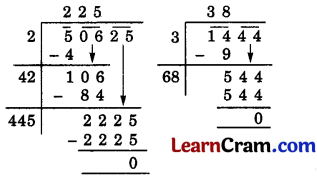= $$\frac{225}{38}=5 \frac{35}{38}$$Question 2.
(i) $$\sqrt{0.0009}$$
(ii) $$\sqrt{0.0081}$$
(iii) $$\sqrt{0.012321}$$
(iv) $$\sqrt{7.29}$$
Solution:
(i) $$\sqrt{0.0009}$$Hence $$\sqrt{0.0009}$$ = 0.03

(ii) $$\sqrt{0.0081}$$Hence $$\sqrt{0.0081}$$ = 0.09

(iii) $$\sqrt{0.012321}$$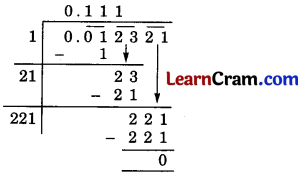Hence $$\sqrt{0.012321}$$ = 0.111

(iv) $$\sqrt{7.29}$$Hence $$\sqrt{7.29}$$ = 2.7.Question 3.
Find the square root of
(i) 0.053361
(ii) 0.00053361
(iii) 150.0625
(iv) 0.374544
(v) 610.09
Solution:
(i) 0.053361Hence $$\sqrt{0.053361}$$ = 0.231

(ii) 0.00053361Hence $$\sqrt{0.00053361}$$ = 0.231

(iii) 150.0625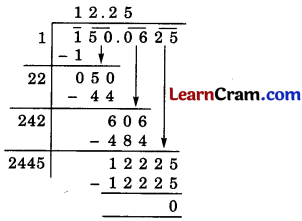Hence $$\sqrt{150.0625}$$ = 12.25

(iv) 0.374544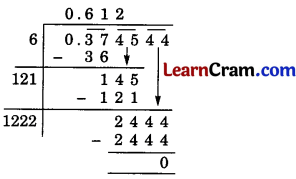Hence $$\sqrt{0.374544}$$ = 0.612

(v) 610.09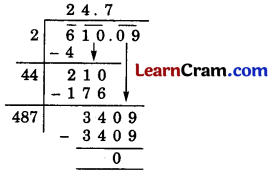Hence $$\sqrt{610.09}$$ = 24.7Question 4.
Find the square root of the following (correct to three decimal places).
(i) 7
(ii) 2.5
(iii) $$2 \frac{1}{12}$$
(iv) 367 $$\frac{2}{7}$$
Solution:
(i) 7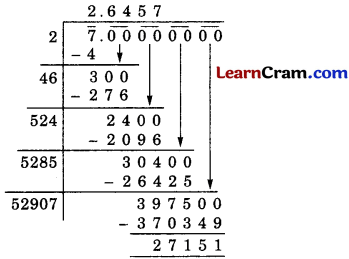Hence $$\sqrt{7}$$ = 2.6457
= 2.646 (correct to 3 places of decimal)

(ii) 2.5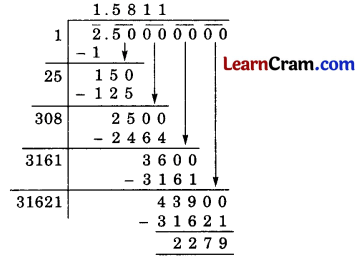Hence $$\sqrt{2.5}$$ = 1.5811
= 1.581 (correct to 3 places of decimal)

(iii) $$\sqrt{2 \frac{1}{12}}=\sqrt{\frac{25}{12}}=\sqrt{\frac{5^2}{12}}=\frac{5}{\sqrt{12}}$$Hence, $$\sqrt{2 \frac{1}{12}}=\frac{5}{3.464}$$ = 1.443

(iv) 367 $$\frac{2}{7}$$
$$\sqrt{367 \frac{2}{7}}=\sqrt{\frac{2571}{7}}$$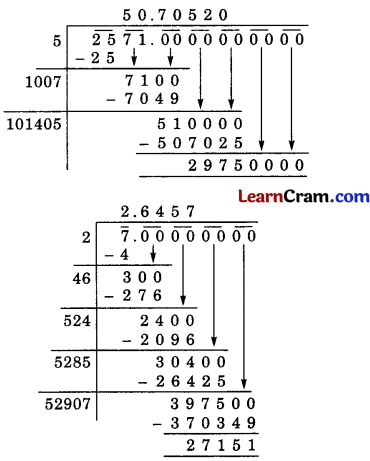Hence, $$\sqrt{367 \frac{2}{7}}=\frac{50.705}{2.646}$$
= 19.163 (correct to three places of decimal)Question 5.
Estimate the value of the following to the nearest one decimal place.
(i) $$\sqrt{90}$$
(ii) $$\sqrt{150}$$
(iii) $$\sqrt{600}$$
(iv) $$\sqrt{1000}$$
Solution:
(i) The perfect square near 90 are 81 and 100.
∴ 81 < 90 < 100
⇒ 92 < 90 < 102
So we can estimate the value between 9 and 10. Let us try with 9.5.
∴ 9.5 × 9.5 = 90.25 > 90
∴ 92 < 90 < (9.5)2
Now 9.4 × 9.4 = 88.36 < 90
Hence, the estimated value of $$\sqrt{90}$$ is 9.48.

(ii) The perfect square number near 150 are 144 and 169.
∴ 144 < 150 < 169
⇒ 122 < 150 < 132
So we can estimate the value of $$\sqrt{150}$$ between 12 and 13.
Let us try for 12.5.
∴ 12.5 × 12.5 = 156.25 > 150
and 12.4 < 12.4 = 153.76 12.3 × 12.3 = 151.29 > 150
12.2 × 12.2 = 148.84 < 150
∴ 12.22 < 150 < 12.42
∴ 122 < 150 < 12.52
∴ 122 < 150 < 12.42
∴ 122 < 150 < 151.29
∴ 148.84 < 150 < 151.29
∴ Estimated value of $$\sqrt{150}$$ = 12.25.

(iii) The perfect squares near 600 are 576 and 625.
∴ 576 < 600 < 625
⇒ 242 < 600 < 252
Let us try for 24.5.
∴ 24.5 × 24.5 = 600.25 > 600
∴ 242 < 600 < 24.52
24.4952 = 600.005
Hence, the estimated value of $$\sqrt{600}$$ = 24.495

(iv) The perfect square numbers near 1000 are 961 and 1024.
∴ 961 < 1000 < 1024
⇒ 312 < 1000 < 322
Let us try for 31.5
∴ 31.5 × 31.5 = 992.25 < 1000
and 31.6 × 31.6 = 998.56 < 1000 then 31.7 × 31.7 = 1004.89 > 1000
∴ 31.62 < 1000 < 31.72
31.622 = 999.82 < 1000
Hence, the estimated value of $$\sqrt{1000}$$ is 31.63.Question 6.
Devika has a square piece of cloth of area 9 m2 and she wants to make 16 square-shaped scarves of equal size out of it. What should possibly be the length of the side of the scarf that can be made out of this piece?
Solution:
Area of 1 square shapped scarf = $$\frac{9}{16}$$ m2

side of the scarf = $$\sqrt{\frac{9}{16}}=\frac{\sqrt{9}}{\sqrt{16}}$$
= $$\frac{\sqrt{3^2}}{\sqrt{4^2}}=\frac{3}{4}$$ m.

Hence, the length of the scarf = $$\frac {3}{4}$$ m.

Question 7.
The area of a square plot is 800 m2. Find the estimated length of the side of the plot.
Solution:
The perfect square numbers near to 800 are 784 and 841.
∴ 784 < 800 < 841
⇒ 282 < 800 < 292
Let us check for 28.5.
∴ 28.5 × 28.5 = 812.25 > 800
∴ 282 < 800 < 28.52
28.4 × 28.4 = 806.56 < 800
∴ 282 < 800 < 28.4
28.3 × 28.3 = 800.89
∴ 282 < 800 < (28.3)2
28.2 × 28.2 = 79.524 < 800
∴ 28.22 < 800 < 28.32
∴ Estimated value of $$\sqrt{800}$$ = 28.25
Hence, the length of the side of the plate = 28.25 m### DAV Class 8 Maths Chapter 1 Value Based Questions

Question 1.
Priya wants to wish her teacher on Teacher’s Day by giving her a self-made greeting card. She chooses a pink coloured square sheet of paper. A side of that paper measures 19.5 cm.
(i) Find the area of paper she chooses for the card.
(ii) What act of Priya did you like?
Solution:
(i) ∵ Side of square sheet of paper she chooses for the card = 19.5 cm
∴ Area of the card = (side)2 = (19.5 cm)2
= 19.5 cm x 19.5 cm
= 380.25 cm2

(ii) Respect, gratitude and thankful nature.

Question 2.
The students of Class-VIII B of a school donated 2,304 for the Prime Minister’s National Relief Fund. Each student donated as many rupees as the number of students in the class.
(i) Find the number of students in VIII B.
(ii) What quality of the students do you appreciate here?
Solution:
(i) Total donation = 2,304
∴ No. of students in class VIII B = $$\sqrt{2,304}$$
= $$\sqrt{48 \times 48}$$ = 48.

(ii) The students exhibit responsibility and duty towards the nation.### DAV Class 8 Maths Chapter 1 Worksheet 5 Notes

Square root of a rational number:

(i) $$\sqrt{a \times b}=\sqrt{a} \times \sqrt{b}$$
(ii) $$\sqrt{\frac{a}{b}}=\frac{\sqrt{a}}{\sqrt{b}}$$, b ≠ 0

Example 1.
Find $$\sqrt{36 \times 81}$$ and show that $$\sqrt{36 \times 81}=\sqrt{36} \times \sqrt{81}$$
Solution:
$$\sqrt{36 \times 81}$$ = $$\sqrt{6^2 \times 9^2}$$
=$$\sqrt{(6 \times 9)^2}$$
= $$\sqrt{(54)^2}$$
= 54
Now $$\sqrt{36} \times \sqrt{81}=\sqrt{6^2} \times \sqrt{9^2}$$
= 6 × 9 = 54
Hence, $$\sqrt{36 \times 81}=\sqrt{36} \times \sqrt{81}$$

Example 2.
Find $$\sqrt{\frac{49}{121}}$$ and show that $$\sqrt{\frac{49}{121}}=\sqrt{\frac{49}{121}}$$.
Solution:
$$\sqrt{\frac{49}{121}}$$
= $$\sqrt{\frac{7^2}{11^2}}$$
= $$\sqrt{\left(\frac{7}{11}\right)^2}$$
= $$\frac{7}{11}$$
Now $$\frac{\sqrt{49}}{\sqrt{121}}=\frac{\sqrt{7^2}}{\sqrt{11^2}}$$
= $$\frac{7}{11}$$
Hence, $$\sqrt{\frac{49}{121}}=\sqrt{\frac{49}{121}}$$

Example 3.
Find the square root of 1$$\frac{123}{361}$$.
Solution:
$$\sqrt{1 \frac{123}{361}}$$
= $$\sqrt{\frac{484}{361}}$$
= $$\frac{\sqrt{484}}{\sqrt{361}}$$
= $$\frac{\sqrt{22^2}}{\sqrt{19^2}}$$
= $$\frac{22}{19}$$
[∵ $$\sqrt{\frac{a}{b}}=\frac{\sqrt{a}}{\sqrt{b}}$$]Squares of terminating decimals:

Example 4.
Find the squares of the following rational numbers:
(i) 0.32
(ii) 0.2
(iii) 0.008
Solution:
(i) (0.32)2 = 0.1024
(ii) (0.25)2 = 0.0625
(iii) (0.008)2 = 0.000064
(iv) (0.0001)2 = 0.0000001

Square roots of numbers in decimal form (which are perfect squares):

Example 5.
Find the square root of the following decimal numbers:
(i) 17.64
(ii) 147.1369
Solution:

(i)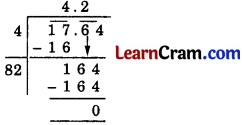Hence, $$\sqrt{17.64}$$ = 4.2

(ii)Hence, $$\sqrt{147.1369}$$ = 12.13.

Approximate value of the square root of natural numbers (Which are not perfect Squares)Example 6.
Find the square root of 5 upto three decimal places.
Solution: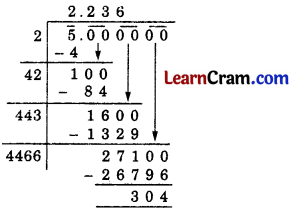Hence, √5 = 2.236 upto 3 decimal place.

Example 7.
Find the square root of 3$$\frac{3}{5}$$ correct to two places of decimals.
Solution:
3$$\frac{3}{5}$$ = $$\frac{18}{5}$$ = 3.6
∴ $$\sqrt{3 \frac{3}{5}}=\sqrt{3.6}$$Hence, $$\sqrt{3 \frac{3}{5}}$$ = 1.897 = 1.90